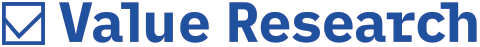# ING Vysya Dynamic Asset Allocation Fund

ING Vysya has come out with the Dynamic Asset Allocation Fund whereby mathematically based computations will determine the asset allocation while the fund manager determines the security selection. So the allocation to debt and equity is determined by an algorithm while the security selection is subjective.
According to the fund house, the rationale behind this structured product is that 60 per cent of the returns are actually determined by smart asset allocation while the balance is determined by stock selection. So the purpose of the fund is to use smart asset allocation to protect downside in a falling market by reducing equity exposure, and to give higher returns by increasing exposure in a rising market.

How it works
The algorithm is based on three parameters: Floor / Multiplier / Rebalancing frequency.
The rebalancing will take place either on a time frame basis or be trigger based. This means that every 15 days, the algorithm will throw up the new percentage of equity and debt exposure. However, there is also the possibility of the market moving during this time frame. So should the market move up or down by 5 per cent consecutively over a few days, then rebalancing is activated, irrespective of whether or not the 15 day time frame has been met.
Based on the parameters of the fund, the Multiplier has been fixed at 3.
On any rebalancing day, the Floor will be the discounted value of initial investment amount at maturity. Let's take Rs 100. The discounted value of Rs 100 at maturity (after three years) is Rs 78. This has been calculated by taking the three-year, AAA paper which is currently at 8.5 per cent. So if you invest Rs 78 in debt now, you will get Rs 100 at maturity.
Let's say the investor invests Rs 100 now. Here's how the allocation is done between equity and debt.

Equity portion
= (Mkt value - Floor) x Multiplier
= (100 - 78) x 3
= 22 x 3
= 66%

Debt portion
= Mkt value - Equity portion
= 100 - 66
= 34%

Assume that the next rebalancing is done after 15 days when the new Floor will not be calculated with three-year paper but two-year, 11 month, 15-day paper. So the Floor would now be 79 (the current discounted value of 100).
Let's also assume that the equity portion grows to 70 per cent and the debt stays at 34 per cent.

Equity portion
= (Mkt value - Floor) x Multiplier
= (104 - 79) x 3
= 25 x 3
= 75%

Debt portion
= Mkt value - Equity portion
= 104 - 75
= 29%

How reliable is it?
This model has been back tested from January 1992 onwards for a three-year period. This was done starting every single month from then onwards and three years were calculated from every start. The Nifty was used as proxy for active fund management.
The absolute returns revealed that when the Nifty rose, the returns generated from such a product also rose but by a lesser margin. But when the Nifty crashed, this one fell by much less.
When the Nifty gave an absolute return of 280.88 per cent, the dynamic asset allocation model gave one of 259.99 per cent (May 2003 - April 2006). But when the Nifty gave an absolute negative return of (-) 35.74 per cent, the dynamic model gave one of 0.95 per cent (March 2000 - February 2003).

Where will the money be invested?
With the universe restricted to the BSE 100 for large-caps and the BSE 500 for other stocks, the fund manager will have the flexibility to move between mid-, small-, and large-caps, depending on the market condition.
In a situation where the exposure to equity is decreasing (falling market), then exposure to large-caps would increase.
Where debt is concerned, high credit short tenor instruments which provide high liquidity and negligible interest rate risk will be preferred. But if debt exposure is increasing and the equity portion is almost nil, then high credit instruments whose maturity matches that of the fund will be selected.
The fund has been rated AAA (so) by CRISIL, indicating highest degree of certainty regarding payment of initial investment to unit holders. The credit quality of the paper, the rebalancing, the selection of stocks from the designated universe and all other specifications will be monitored by CRISIL.

Snapshot
Offer opened: September 27, 2006
Offer closes: October 17, 2006